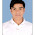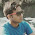साझेदारी: Maths Partnership Problems Short Tricks in Hindi साझेदारी शार्ट ट्रिक्स Partnership Short Tricks - Online Solution, Maths Short Tricks, Educational, Mobile Features, Toll Free, customer care number

# साझेदारी: Maths Partnership Problems Short Tricks in Hindi साझेदारी शार्ट ट्रिक्स Partnership Short Tricks

## Partnership Short Tricks साझेदारी शार्ट ट्रिक्स

In this article we serve most important and useful short tricks of partnership questions in Hindi as well as English. It is very useful tricks for those candidates who want to crack various national level and state level emulative examinations. In this article we also share some most important questions which asked in frequently in the exam.

## Introduction:

Partnership (साझेदारी) :-
A partnership is an arrangement where parties, known as partners, agree to cooperate to advance their mutual interests. The partners in a partnership may be individuals, business, interest-based organization, school, government or combinations.

Capital (पूंजी) :
The money, property , and other valuables which collectively represent the wealth of an individual or business.

Type and Partnership Short trick 1:-

If both A and B invested capital for same time, then:-
Profit of A (Pa) Profit of B (Pb) = Capital of A (Ca) : Capital of B(Cb).

Example :- X and Y together started a business. In the end of year they divided the profit into ratio of 2:3.If X invested 40000 rs , then who much wealth did Y invested?

Most Important Questions (Asked in Exam)

 Ex 1. 4. A, B and C enter into partnership. A invests 3 times as much as B invests and B invests two-third of what C invests. At the end of the year, the profit earned is Rs. 6600. What is the share of B? A , B और C तीनों पार्टनर्शिप करते है। A b से 3 गुना ज्यादा पेसे लगता है और B 2/3 लगता है सी के पेसो से। साल खतम होने के बाद 6600rs का लाभ होता है। बी का हिस्सा बताए। Sol. Let C’s capital = Rs. x. Then, B’s capital = Rs. 2x/3 A’s capital Rs. (3x2x/3) = Rs. 2x .. Ratio of their capitals = 2x: 2x/3 : x = 6: 2: 3. Hence, B’s share —> Rs. (6600 x 2/11)  = Rs. 1200. Ex 2. Four milkmen rented a pasture. A grazed 24 cows for 3 months; B 10 cows for 5 monthr, C 35 cows for 4 months and D 21 cows for 3 months. If A’s share of rent is Re. 720, find the total rent of the field. 4 किसानो ने एक बाड़ा किराए से लिया। यदि A की 24 गाए 3 महीने के लिए ; B की 10 गाए  5 महीनो के लिए, C की 35 गाए 4 महीनो के लिए और D की 21 गाए  3 महीनो के लिए बांधते है। A 720rs किराया देता है तो टोटल किराया बताए Sol. Ratio of shares ofA, B, C, D = (24x3) : (10x5): (35x4) : (21x3) -> 72: 50 : 140: 63. Let total rent be Rs. x. Then, A’s share = Rs. 72x/325 72x/325 = 720 x = 720 x 325 / 72 = 3250 Hence, total rent of the field is Rs. 3250. Ex. 3. A invested Rs. 76,000 in a business. After few months, B joined him with Re. 57,000. At the end of the year, the total profit was divided between them in the ratio 2: 1. After how many months did B join? A 76000rs एक व्यापार मे लगता है। कुश महीनो बाद B व्यापार मे आता है और 57000rs लगता है। साल के अंत मे वो लाभ को 2:1 मे बाटते है। B ने कब व्यापार मे आया। Sol. Suppose B joined after x months. Then, B’s money was invested for (12 — x) months. (76000x12) / (57000x(12-x)) = 2/1 = 912000 = 114000 (12 — x) 114(12—x)=912  (12—x)=8 x=4, Hence, B joined after 4 months. Ex. 4. A, B and C enter into a partnership by investing in the ratio of 3: 2: 4. Afterone year, B invests another Rs. 2,70,000 and C, at the end of 2 years, also invests Rs. 2,70,000. At the end of three years, profits are shared in the ratio of 3: 4: 5. Find the initial investment of each. A , B और C एक व्यापार मे 3:2:4 मे पेसे लगते है। एक साल बाद B 2,70,000rs और लगता है तथा c भी  2 साल बाद 270000rs लगता है। 3 साल बाद वो लाभ को 3:4:5 मे बाटते है। तीनों का प्रारम्भ का हिस्सा बताए Sol. Let the initial investments of A, B and C be Rs. 3x, Rs. 2x and Rs. 4x respectively. Then, (3x x 36) : [(2x x 12) + (2x + 270000) x 24] : [(4x x 24) + (4x + 270000) x 12] = 3 : 4 : 5. 108x : (72x + 6480000) : (144x + 3240000) = 3 : 4 : 5 108x / (72x + 6480000) = 3/4  = 432x = 216x + 19440000 216x = 19440000 x = 90000. Hence, A’s initial investment = 3x = Rs. 2,70,000; B’s initial investment = 2x = Rs. 1,80,000; C’s initial investment = 4x = Rs. 3,60,000.

Type and Partnership Short trick 2:-

If A , B and C invested capital for same time , then:-
Profit of A (Pa) : Profit of B(Pb): Profit of C (Pc) = Capital of A (Ca) : Capital of B (Cb) : Capital of C (Cc)

Example :- Deepak , Ram and Amar invested 2700 rs , 8100 rs and 7200 rs and started a business. In the end of year Ram got profit of 3600 rs , find there total profit.

Type and Partnership Short trick 3:-

If A and B invested different capital for different time , then:-
Profit of A (Pa) : Profit of B(Pb) = Capital of A x time of A (Ca x Ta) : Capital of B x time of A (Cb x Tb)
or
Pa/Pb = (Ca x Ta) / (Cb x Tb)
Example :- Rajan invested 15000 rs and started a business. After 3 month Ajay invested 45000 rs and jointed the business. If in the end of year profit of 5850 rs will gained, then find difference between profit og Ajay and Rajan.

Type and Partnership Short trick 4:-

If A ,B and C invested different capital for different time , then:-
Profit of A (Pa) : Profit of B(Pb): Profit of C (Pc) = Capital of A x time of A (Ca x Ta) : Capital of B x time of A (Cb x Tb): Capital of C x time of C (Cc : Tc)

Example :- A and B invested 12000 rs and 16000 rs and started a business. After 9 months C also invested 15000 rs and join the business. After 2 years they bought profit of 45600 rs, find the profit of C.

Type and Partnership Short trick 5:-

If A and B get a grassland on rent, A graze X cows for time T1 and B graze Y cows for time T2 , then:-
Rent of A / Rent of B = (X x T1) / (Y x T2)

Example :- A and B get a grassland on rent of 30 rs/month for 10 months. If A's 10 cows graze for 9 months, then how much cows should B graze so that he has to pay 60 rs less rent then A?

Type and Partnership Short trick 6:-

If A , B and C get a video kyset on rent of X rs and they used it for T1 hours , T2 hours and T3 hours, then :-

1. Rent should be given by A = [{T1 / (T1+T2+T3)} x X] rs
2. Difference between the rent should be paid by B and C = [{(T2-T3) / (T1+T2+T3)} x X] rs                          ,[there T2 > T3]

Example :- Nayar , Mani and Lona get a video shouting unit on rent of 9800 rs for one week. They sequently used that unit for 12 hours , 6 hours and 10 hours. How much rent Nayar had to pay ?

Type and Partnership Short trick 7:-

Example :- A, B and C together started a business. A invested 1/3 part of total capital and B invested amount that A and C together invested . In the end of year how much profit did C got from total profit of 18000 rs ?

Type and Partnership Short trick 8:-

Example :- A and B together started a business and invested capital in ratio of 4:5. After 3 months A take bake 1/4 part of his investment and B takes 1/5 part of hos investment. After 10 months of starting they got profit of 3800 rs ,how much money did B got from the profit?

Type and Partnership Short trick 9:-

Example :- A invested 1200 rs and started a business. B and C invested different amount of capital after 3 months and 6 months sequentially and join the business.  If in the end of month they divided the profit into ratio of 2:3:5 , then how much capital did B invested?

Type and Partnership Short trick 10:-

Example :- Kiran and Nandan together started a business. Kiran invested thrice more capital then Nandan for double of time. If from the total profit Nandan got 4000 rs, then find the total profit.

Type and Partnership Short trick 11:-

Example :- A and B sequentially invested 15000 rs and 12000 rs and started a business. A is a effectual partner so he got 12(1/2)% of total profit. The remaining amount of profit both of them got as the ratio of there investment. If 2160 rs is total profit, then how much profit did A got?

Type and Partnership Short trick 12:-

Example :- A, B and C are three partners. A got 2/3 part of total profit. The remaining profit is divide equally between B and C. Profit of A increased by 200 rs when total profit increase from 5% to 7%. Find the capital of B.

Type and Partnership Short trick 13:-

Example :- A, B and C invested capital for same time in such way that if A got 2 rs profit then B will get 3 rs and if B got 5 rs then C will get 4 rs of profit. Such that B get 3000 rs from the profit, then how much profit did A and C will get?

## People also search Related with: Aptitude in Hindi, Maths small  Tricks work and Time, cut,  Tricks for PSC,  Tricks for  , small  puzzles with answers,  tricks of ,  tricks of  in hindi,  tricks of  pdf,  tricks of  for ,  tricks of  for bank po exam,  tricks of  for ,  tricks of  for competitive exam,  Small Tricks, small  puzzles with answers,  tricks of ,  tricks of  in hindi,  tricks of  pdf,  tricks of  for ,  tricks of  for bank po exam,  tricks of  for  ,  tricks of  for competitive exam,  Formulas  Problems,  tricks Objectives,  Objectives tricks, problems and formulas,  Objectives questions with  tricks,  method to find ,  to calculate,   tricks pdf,   tricks in hindi,  tricks for cat,  Objectives formulas,  Objectives problems with solutions pdf, how to do  problems,  to find,  method to find ,  s pdf,  to find of three numbers,   tricks in hindi, how to calculate ,  formulas,  Tricks  Problems MAT Objectives ,  Tricks  Problems CDSE ,  Tricks  Problems SBIClerk ,  Tricks  Problems SBIPO ,  Tricks  Problems IBPSClerk ,  Tricks  Problems IBPSPO ,  Tricks  Problems IBPS ,  Tricks  Problems RRB ,  Tricks  Problms Objectives CGLTier 1 ,  Tricks  Problems CGL Tier 2 ,  Tricks  Problems  10+2 ,  Tricks  Problems CLAT ,  Tricks  Problems NIFT ,  Tricks  Problems SBI ,  Tricks  Problems PO Pre ,  Tricks  Problems IBPS PO Pre ,  Tricks  Problems IBPS Clerk Pre Objectives ,  Tricks  Problems CET , Short, mcqs with answers pdf, competitive exams free download, quiz questions with answers pdf, objective questions, in hindi, , vyapam, railway, Bank PO Clerk, NET, UGC, CSIR, BEd, TET, CTET, Navodaya, Central School,  Model Question Paper, Online Practice e Test, Samvida, Teachers, Download, Syllabus, ओल्ड पेपर्स , ssc, Download, Previous year questions and solution

1.Hi Sir Which Book You Capture This Photo Tell Me Book Name

2.Sir ye question kis bool se h plz book ka bataye

3.Jo cutting hain book ki us book ka name bataye sir plz

4.Sir book ka name bataye plz jiski cutting apne dali h

5.Top